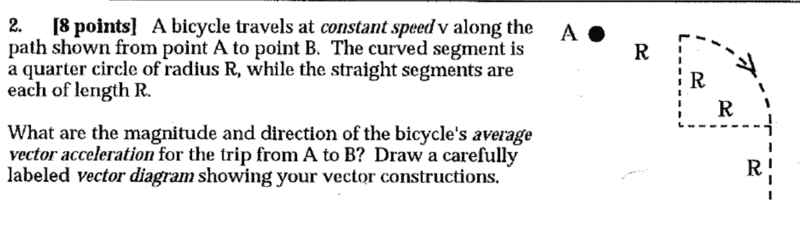# Average Vector Acceleration Along a Curve and a Straight Section

• srekai
Average Acceleration is simply the magnitude of the difference between the final and initial velocity vectors.

## Homework Statement

ff

##a = {v^2}{r}##
##d = vt##

## The Attempt at a Solution

The way I do it is break it down into the curved and straight segment.
The bicycle is always traveling at a constant speed.
Curved portion:
distance ##\frac{\pi R^2}{4}##
acceleration ##= \frac{v^2}{R}##
time ##t = \frac{\frac{\pi R^2}{4}}{v}##

Straight portion:
distance ##R##
acceleration 0
time ##t = \frac{R}{v}##

Combining these two pieces
avg acc. = ##\frac{\frac{v^2}{R} \cdot \frac{\pi R^2}{v}}{\frac{\pi R^2}{v} +\frac{R}{v}} = \frac{\pi Rv}{\frac{\pi R^2+R}{v}} = \frac{\pi v^2}{\pi R + 1}##

As for the direction, I'm assuming it's to the right?

#### Attachments

Since the acceleration around the arc is always pointing at the center of the circle, what would be the average direction on that part? Then there is no acceleration in the straight part, so the direction is not changed.

PS. When you use the term "right" you should be clear if you are talking about the right of the diagram or to the right of the velocity vector forward direction.

srekai said:
##a = {v^2}{r}##
I assume you meant v2/r. That is the magnitude of the acceleration. You are told to average acceleration as a vector.
What is the definition of average acceleration?
srekai said:
distance ##\frac{\pi R^2}{4}##
That is an area, not a distance.

•FactChecker
srekai said:

## Homework Statement

fView attachment 229300
f

##a = {v^2}{r}##
##d = vt##

## The Attempt at a Solution

The way I do it is break it down into the curved and straight segment.
The bicycle is always traveling at a constant speed.
Curved portion:
distance ##\frac{\pi R^2}{4}##
acceleration ##= \frac{v^2}{R}##
time ##t = \frac{\frac{\pi R^2}{4}}{v}##

Straight portion:
distance ##R##
acceleration 0
time ##t = \frac{R}{v}##

Combining these two pieces
avg acc. = ##\frac{\frac{v^2}{R} \cdot \frac{\pi R^2}{v}}{\frac{\pi R^2}{v} +\frac{R}{v}} = \frac{\pi Rv}{\frac{\pi R^2+R}{v}} = \frac{\pi v^2}{\pi R + 1}##

As for the direction, I'm assuming it's to th
srekai said:

## Homework Statement

fView attachment 229300
f

##a = {v^2}{r}##
##d = vt##

## The Attempt at a Solution

The way I do it is break it down into the curved and straight segment.
The bicycle is always traveling at a constant speed.
Curved portion:
distance ##\frac{\pi R^2}{4}##
acceleration ##= \frac{v^2}{R}##
time ##t = \frac{\frac{\pi R^2}{4}}{v}##

Straight portion:
distance ##R##
acceleration 0
time ##t = \frac{R}{v}##

Combining these two pieces
avg acc. = ##\frac{\frac{v^2}{R} \cdot \frac{\pi R^2}{v}}{\frac{\pi R^2}{v} +\frac{R}{v}} = \frac{\pi Rv}{\frac{\pi R^2+R}{v}} = \frac{\pi v^2}{\pi R + 1}##

As for the direction, I'm assuming it's to the right?
Surely Average Acceleration is simply (final Velocity - initial velocity)/time taken.
In this case the subtraction is the subtraction of two vectors, and you have to work out an expression for the time interval, using speed and distance.

•FactChecker
PeterO said:
Surely Average Acceleration is simply (final Velocity - initial velocity)/time taken.
In this case the subtraction is the subtraction of two vectors, and you have to work out an expression for the time interval, using speed and distance.
I think that this is what @haruspex was (more subtly) alluding to.

•haruspex